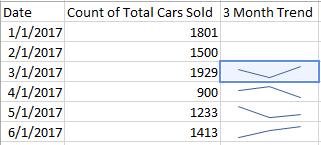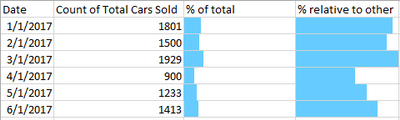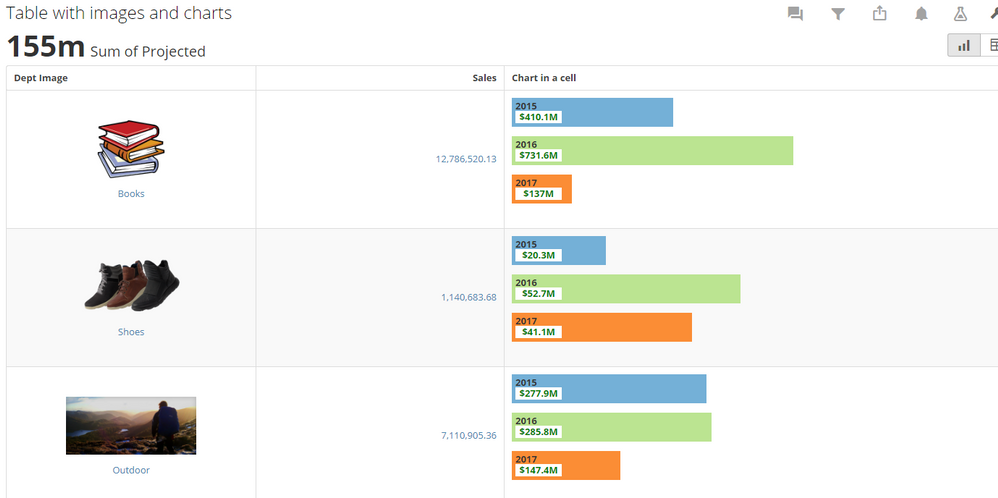# Extend Table Card Functionality (Graphics)

It would be nice if you could input little graphs into a table such that you can see a bar graph, line graph, etc in a cell which adds a little more meaning to the data it's representing.

It would be VERY cool if you could include some dynamic info based on if the cell is aggregated or not.

Ex.Maybe the ability to set a value or use the largest value available to show what 100% is and fill the cell with color a % representation of the total/100% value

Ex. (Mockup so % bars not necessarilly accurate)**Make sure to like any users posts that helped you and accept the ones who solved your issue.**
24

#### · Last Updated January 2021

• Thank you for submitting this @guitarhero23. I am assigning to our product manager @Gavatar to review and comment.

• We are working at ways to make this easier but for now there is a "beast mode" way to create a small bar chart in your table.  The key is to generate HTML "div" statements in your table with width and fills.  Here is an example table I created:This is the beast mode I use:

CONCAT('<div style="height:170px;width:100%;">
CASE WHEN
SUM(CASE WHEN YEAR(`Ad Start Date`) = 2015 THEN `Sales` ELSE 0 END) = 0
THEN 0
ELSE ROUND(((SUM(CASE WHEN YEAR(`Ad Start Date`) = 2015 THEN `Sales` ELSE 0 END)/SUM(`Sales`))*100),0)
END,
CASE WHEN
SUM(CASE WHEN YEAR(`Ad Start Date`) = 2015 THEN `Sales` ELSE 0 END) = 0
THEN 0
ELSE ROUND((SUM(CASE WHEN YEAR(`Ad Start Date`) = 2015 THEN `Sales` ELSE 0 END)/1000000)*100,1) END,
'M</div></div><br/>',
CASE WHEN
SUM(CASE WHEN YEAR(`Ad Start Date`) = 2016 THEN `Sales` ELSE 0 END) = 0
THEN 0
ELSE ROUND(((SUM(CASE WHEN YEAR(`Ad Start Date`) = 2016 THEN `Sales` ELSE 0 END)/SUM(`Sales`))*100),0)
END,
CASE WHEN
SUM(CASE WHEN YEAR(`Ad Start Date`) = 2016 THEN `Sales` ELSE 0 END) = 0
THEN 0
ELSE ROUND((SUM(CASE WHEN YEAR(`Ad Start Date`) = 2016 THEN `Sales` ELSE 0 END)/1000000)*100,1) END,
'M</div></div><br/>',
CASE WHEN
SUM(CASE WHEN YEAR(`Ad Start Date`) = 2017 THEN `Sales` ELSE 0 END) = 0
THEN 0
ELSE ROUND(((SUM(CASE WHEN YEAR(`Ad Start Date`) = 2017 THEN `Sales` ELSE 0 END)/SUM(`Sales`))*100),0)
END,
CASE WHEN
SUM(CASE WHEN YEAR(`Ad Start Date`) = 2017 THEN `Sales` ELSE 0 END) = 0
THEN 0
ELSE ROUND((SUM(CASE WHEN YEAR(`Ad Start Date`) = 2017 THEN `Sales` ELSE 0 END)/1000000)*100,1) END,
'M</div></div>'
,'</div>')

• Here is a simpler example of just the single bar:

sum(`Sales`)/100000,
'%',
'">',
'</div>'
)

• Thanks Gavatar for some workarounds. That is quite the beastmode.

**Make sure to like any users posts that helped you and accept the ones who solved your issue.**
• Second example is easier• This is exactly what we needed!  @Gavatar, great example and what a beastmode!  Is there any workaround to generate sparklines as I asked in this post?

I tried to use arrows to present the individual trend & connect them together, but without the reference of value, they don't look like a trend line.  If you can suggest some ideas/workaround, that will be much appreciated.

Now seeing the status as "Approved", I will look forward to the feature being released.

This discussion has been closed.